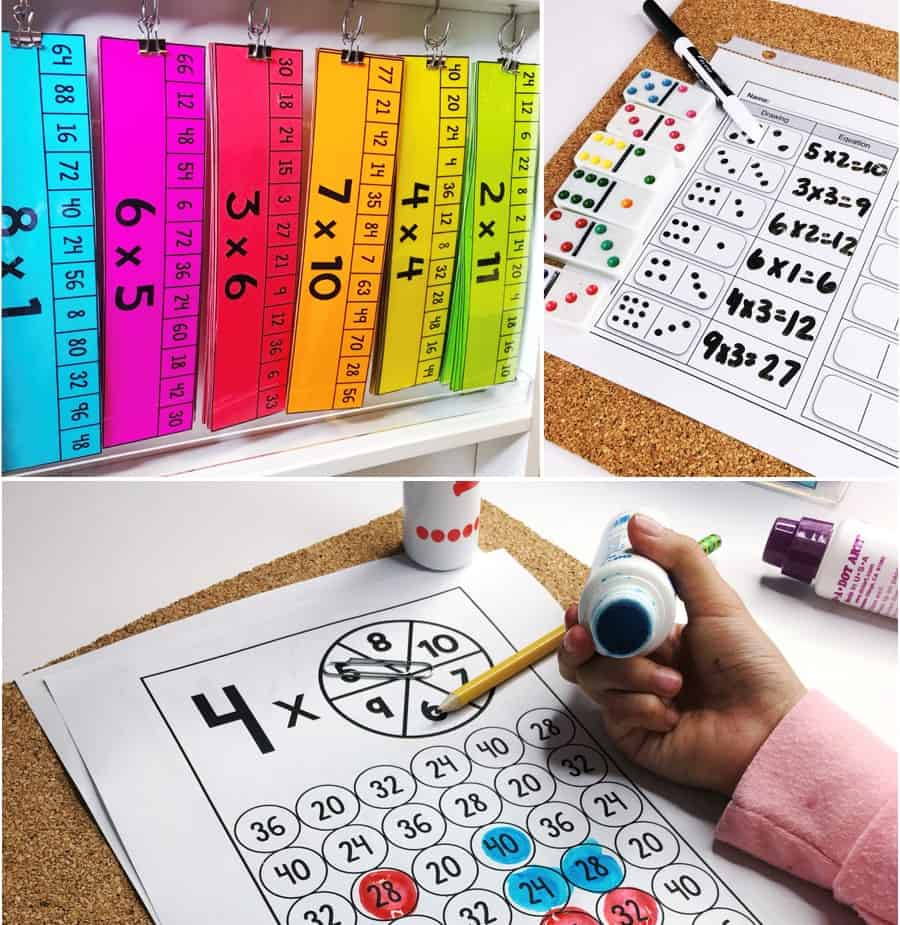Part 1

The Standards for Mathematical Practice & Instructional Materials

What to look for in instructional materials that align to the SMPsPart 2

SMP 1: Make Sense of Problems & Persevere in Solving Them

Well-designed materials help students tackle challenging math problemsPart 3

Reason Abstractly and Quantitatively

A math teacher explains how well-designed materials can help students learn to deal with challenging math problemsPart 4

Digging Deeper into SMP 3 – Construct Viable Arguments and Critique the Reasoning of Others

A math teacher explains how to recognize math tasks that help students craft strong mathematical argumentsPart 5

Putting the Math Practices into Practice

A K-12 math team collaborated in creating tools about the practices to enhance understanding and planningPart 6

Digging Deeper into SMP 4 – Model with Mathematics

Learn the difference between modeling the math and modeling WITH math